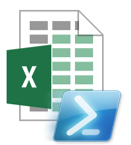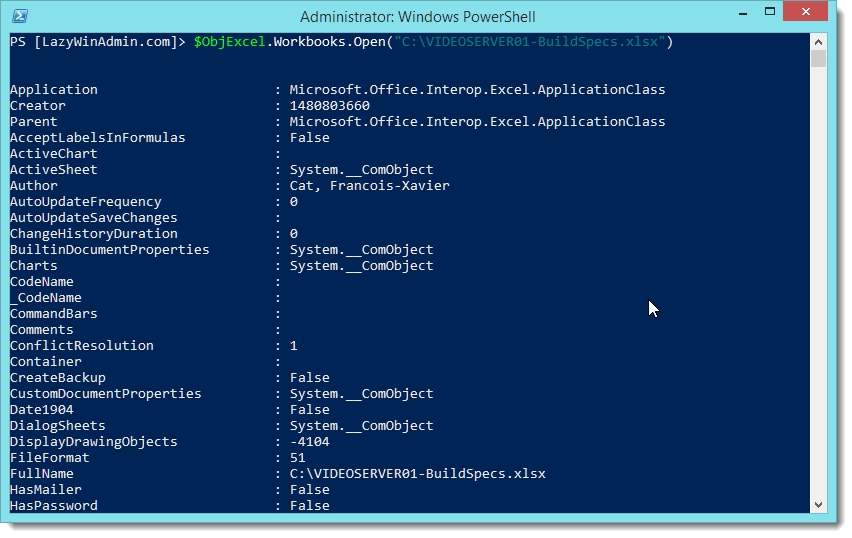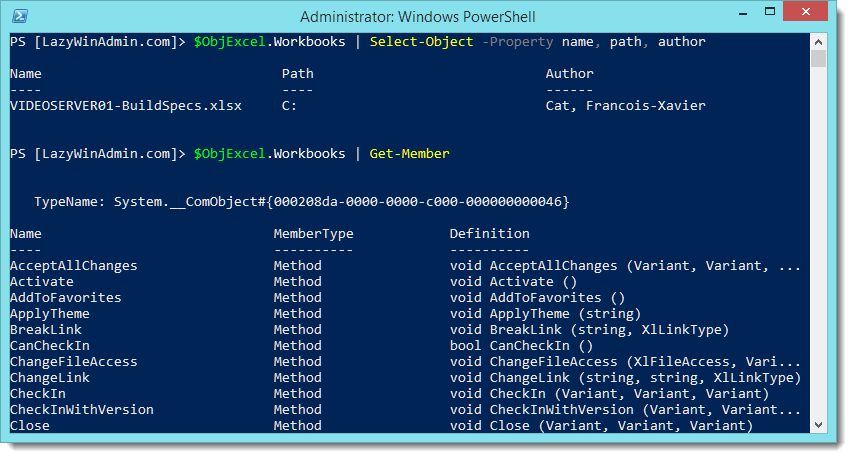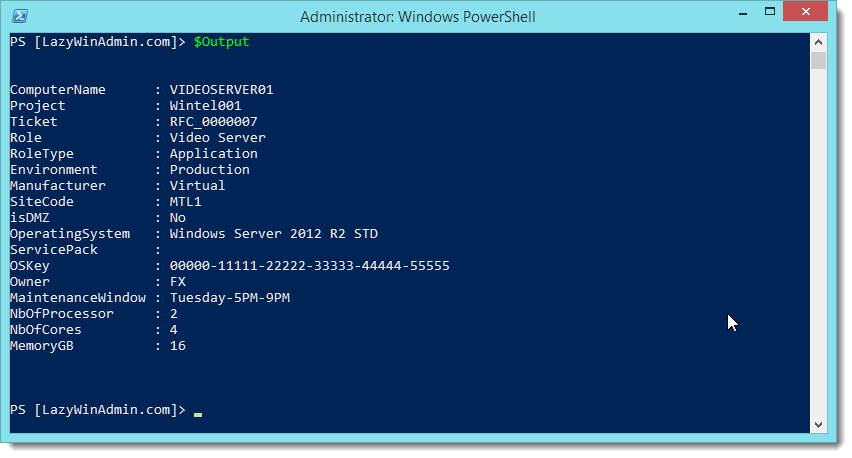# PowerShell - Read an Excel file using COM InterfaceLast week, I worked on a small PowerShell script to read a custom excel file sheet with a lot of information in different columns and rows. Unfortunately, you’ll have to adapt the script to your needs. However I thought this might be helpful to understand how this work.

Here is the sample Excel file I worked with:## COM interface: Excel.Application

Layers To access an Excel file data, you have to be aware of the hierarchy of each elements/layers. The first element will be the application class (at the the top) that contains one or more workbooks, each workbooks contains one or more worksheets, inside each of the worksheet you can access ranges. Each element can access down to some of the other layers.Application layer This will open a new instance of excel on my computer as you can see below:

``````\$objExcel = New-Object -ComObject Excel.Application
``````

MSDN reference: Microsoft.Office.Interop.Excel.ApplicationClassA new Excel Instance is created.

Workbook layer In the second step, we are opening the workbook inside the Excel Instance.

``````\$WorkBook = \$objExcel.Workbooks.Open("C:\VIDEOSERVER01-BuildSpecs.xlsx")
``````We can verify that the workbook is opened using the following line

``````\$objExcel.WorkBooks | Select-Object -Property name, path, author
``````

Then see the properties and methods that can be used

``````\$objExcel.WorkBooks | Get-Member
``````In the following example, I hold the data inside the \$WorkBook variable.

``````\$WorkBook = \$objExcel.Workbooks.Open("C:\VIDEOSERVER01-BuildSpecs.xlsx")
``````

I then look for any member (property, method, or event) that contains the word “sheet”.

``````\$WorkBook | Get-Member -Name *sheet*
``````My WorkBook contains 2 worksheets as you can see in the first image of the article, “BuildSpecs” and “Sheet2”, PowerShell can retrieve this information using the “sheets” property.

``````\$WorkBook.sheets | Select-Object -Property Name
``````WorkSheet layer We select the sheet BuildSpecs and are now able to start working on the data

``````\$WorkSheet = \$WorkBook.sheets.item("BuildSpecs")
``````Loading the file in PowerShell can be done with just a few lines.

``````# Specify the path to the Excel file and the WorkSheet Name
\$FilePath = "C:\VIDEOSERVER01-BuildSpecs.xlsx"
\$SheetName = "BuildSpecs"

# Create an Object Excel.Application using Com interface
\$objExcel = New-Object -ComObject Excel.Application

# Disable the 'visible' property so the document won't open in excel
\$objExcel.Visible = \$false

# Open the Excel file and save it in \$WorkBook
\$WorkBook = \$objExcel.Workbooks.Open(\$FilePath)

\$WorkSheet = \$WorkBook.sheets.item(\$SheetName)
``````

Let’s get the ComputerName value which is `VIDEOSERVER01` is this example. If you look at the sheet “BuildSpecs” below, you’ll see the ComputerName information in the C3 cell. We can also refer to it using the coordinate-systems: Third column/third row.Different methods are possible to retrieve the information from the sheet, I found the following ones:

``````\$worksheet.Range("C3").Text
\$worksheet.Range("C3:C3").Text
\$worksheet.Range("C3","C3").Text
\$worksheet.cells.Item(3, 3).text
\$worksheet.cells.Item(3, 3).value2
\$worksheet.Columns.Item(3).Rows.Item(3).Text
\$worksheet.Rows.Item(3).Columns.Item(3).Text
\$worksheet.UsedRange.Range("c3").Text
``````

Finally we can create a PowerShell object to output the information we want to extract from the Excel file.

``````\$Output = [pscustomobject][ordered]@{
ComputerName = \$WorkSheet.Range("C3").Text
Project = \$WorkSheet.Range("C4").Text
Ticket = \$WorkSheet.Range("C5").Text
Role = \$WorkSheet.Range("C8").Text
RoleType = \$WorkSheet.Range("C9").Text
Environment = \$WorkSheet.Range("C10").Text
Manufacturer = \$WorkSheet.Range("C12").Text
SiteCode = \$WorkSheet.Range("C15").Text
isDMZ = \$WorkSheet.Range("C16").Text
OperatingSystem = \$WorkSheet.Range("C18").Text
ServicePack = \$WorkSheet.Range("C19").Text
OSKey = \$WorkSheet.Range("C20").Text
Owner = \$WorkSheet.Range("C22").Text
MaintenanceWindow = \$WorkSheet.Range("C23").Text
NbOfProcessor = \$WorkSheet.Range("C26").Text
NbOfCores = \$WorkSheet.Range("C27").Text
MemoryGB = \$WorkSheet.Range("C29").Text
}
``````## Script example

Here is an example with all the pieces together.

``````#Specify the path of the excel file

#Specify the Sheet name
\$SheetName = "BuildSpecs"

# Create an Object Excel.Application using Com interface
\$objExcel = New-Object -ComObject Excel.Application
# Disable the 'visible' property so the document won't open in excel
\$objExcel.Visible = \$false
# Open the Excel file and save it in \$WorkBook
\$WorkBook = \$objExcel.Workbooks.Open(\$FilePath)
\$WorkSheet = \$WorkBook.sheets.item(\$SheetName)

[pscustomobject][ordered]@{
ComputerName = \$WorkSheet.Range("C3").Text
Project = \$WorkSheet.Range("C4").Text
Ticket = \$WorkSheet.Range("C5").Text
Role = \$WorkSheet.Range("C8").Text
RoleType = \$WorkSheet.Range("C9").Text
Environment = \$WorkSheet.Range("C10").Text
Manufacturer = \$WorkSheet.Range("C12").Text
SiteCode = \$WorkSheet.Range("C15").Text
isDMZ = \$WorkSheet.Range("C16").Text
OperatingSystem = \$WorkSheet.Range("C18").Text
ServicePack = \$WorkSheet.Range("C19").Text
OSKey = \$WorkSheet.Range("C20").Text
Owner = \$WorkSheet.Range("C22").Text
MaintenanceWindow = \$WorkSheet.Range("C23").Text
NbOfProcessor = \$WorkSheet.Range("C26").Text
NbOfCores = \$WorkSheet.Range("C27").Text
MemoryGB = \$WorkSheet.Range("C29").Text
}
``````